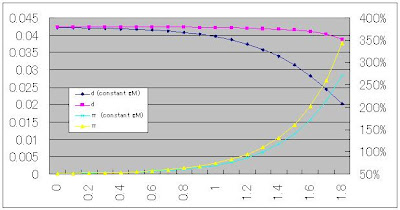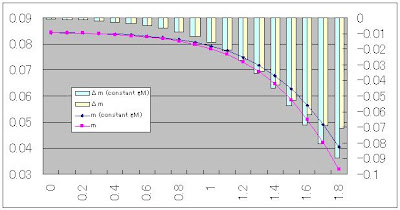# himaginary's blog

## 2010年4月9日金曜日

### A hyperinflation model: circumventing Buiter's critique by holding nominal money growth constant

In hyperinflation model, Cagan money demand function is commonly used (See David Romer, "Advanced Macroeconomics").

m = C・exp(-bπ)

where m is real money stock, π is the rate of inflation, b and C are constant parameters.

Rewriting this as an equation for inflation gives us

π = (-1/b)・ln(m/C)

So, government seigniorage d can be written as

d = Δm + πm
= Δm - (m/b)・ln(m/C)

Therefore,

Δm = d + (m/b)・ln(m/C)

Differentiating this with m gives us

∂Δm/∂m = (1/b)・{ln(m/C) + 1}

Which becomes zero when m = C・exp(-1).

Second derivative of Δm is

∂2Δm/∂m2 = 1/bm

which is strictly positive. So

Δm = d - (C/b)・exp(-1)

is the minimum value of Δm.

Here, d = (C/b)・exp(-1) gives us the maximum value of d presuming Δm=0.

Proof)
∂(πm)/∂π = C・exp(-bπ) - bCπ・exp(-bπ)
= (1-bπ)・C・exp(-bπ)
Therefore, πm, or seigniorage when Δm=0,
becomes maximum when π = 1/b.
At that point,
m = C・exp(-1)
and
d = (C/b)・exp(-1)

So, if we denote this maximum d under stationary condition as d*, the above Δm minimum value becomes

Δm = d - d*

Hyperinflation is thought to happen when d > d*. However, in that case, Δm becomes positive. Which means, m increases, and π＝(-1/b)・ln(m/C) decreases. This situation should be called hyperdeflation, not hyperinflation. This is the paradox noted by Willem Buiter (for detail, see Alexandre Sokic, "Monetary hyperinflation, speculative hyperinflation and modelling the use of money").

And if we think about nominal money growth rate (let's denote this gM) in this hyperdeflation case, it can be shown that

ΔgM / gM = - (Δm / m)

This is because d is assumed to be constant. So, if Δm is positive, ΔgM is negative. In this paradox case, nominal money growth decreases, but as inflation decreases further, real money growth becomes positive.

If you describe people's behavior in this case, it's something like this:
They watch government move and say, "Oh dear, the government is trying to obtain seigniorage beyond possible point. Let's suppress our economic activity and bring inflation down, so that real money stock increases and the government can obtain and maintain that seigiorage. Then, the central bank doesn't need to print more money. It can even print less money."

Obviously, this just cannot happen -- at least, not in the secular world.

In the real world, gM is the only political tool. So, if the government wants more seigniorage, it makes the central bank to increase gM. At any rate, it never lets gM decrease.

Now, let's see what happens if we hold gM constant.

If gM is constant, Δm becomes

Δm = gMm + (m/b)・ln(m/C)

Differentiating this with m gives us

∂Δm/∂m = gM + (1/b)・{ln(m/C) + 1}

Which becomes zero when m = C・exp(-1-bgM).

Second derivative of Δm is

∂2Δm/∂m2 = 1/bm

which is strictly positive. So

Δm = -(C/b)・exp(-1-bgM)

is minimum value of Δm. And this is strictly negative, which means hyperinflation can occur.

And, if gM is constant, we can obtain general solution for π, which is
π = gM + A・exp(t/b)
where A is constant and t denotes time.

Proof)
Cagan money function
m = C・exp(-bπ)
gives us
(dt/dm)/m = -b(dπ/dt)
And seigniorage is
d = Δm + πm = gMm
So, the following differential equation holds
(Note that I use Δm and dm/dt interchangeably).
gM = -b(dπ/dt) + π
Solving this equation gives the above solution.

As noted above, the government would like to increase gM. This constant-gM-solution may be fit for some short period Δt, but it's unlikely to be adequate description for longer period.

However, maybe increasing gM stepwise in this solution can serve as the ad-hoc but tolerable way to describe the hyperinflation. The following graphs are from an example of such a simulation (click to enlarge).(d=left-hand-axis, gM=right-hand-axis)(d=left-hand-axis, π=right-hand-axis)(m=left-hand-axis, Δm=right-hand-axis)

In this simulation, I set b=1/3, C=0.1, Δt=0.1, and gM(initial value)=0.5. Hence, initial d=gM・C・exp(-bgM)=0.0423.
"Constant gM" case is where gM is always 0.5.
In not-constant-gM-case, I increased gM each period so that d becomes approximately constant.

#### 1 件のコメント:

1.I have a simulation of hyperinflation.

http://howfiatdies.blogspot.com/2013/03/simulating-hyperinflation.html

## 自己紹介

This blog is some thoughts on economics by a Japanese non-economist. Translated from my Japanese blog.